Abstract

This article describes the design of a yarn spinning model based on the use of artificial neural networks, as well as the measurements aimed at collecting the data necessary for this model. Partial models of the spinning process were designed for selected essential yarn quality parameters, such as tenacity, yarn hairiness, and yarn faults. Feed-forward neural networks were used for modeling. Yarns manufactured from flax/cotton blended slivers and from a pure cotton sliver with the use of a BD 200S rotor-spinning machine were analyzed. The flax content in the blended slivers was 10, 20, 30, 40, and 50 %. Yarns of linear density of 20, 30, 40, 50, and 60 tex were manufactured from a sliver of linear density of 3 ktex.

Keywords: yarn blends, flax/cotton blends, rotor spinning, yarn quality parameters, artificial neural networks, feed-forward networks.

Introduction

Flax fibres are domestic raw material from which products are manufactured with very good usage parameters, and with resistance to UV radiation.

The investigations presented in this article are a continuation of research work into cotton/flax blended yarns carried out previously by the authors [2, 3].

An analysis of yarns manufactured with the use of a BD 200S rotor-spinning machine from blended flax/cotton slivers and from pure cotton sliver is described below. The rotor-spinning frame was fed with cotton sliver and with blended slivers of the W1 variant, in which flax was used before tearing, and with slivers of the W2 variant in which carded flax was used. The flax content in the blended slivers was of 10, 20, 30, 40, and 50%; the rest of the fibres were of middle-long-staple cotton. The blended slivers of the W2 variant, which included fibres after a more intensive mechanical processing (flax after carding), were characterised by a smaller mass irregularity and higher cohesion as slivers, which included flax with a lower degree of defibration (dividing into the so-called elementary fibres) (W1 - flax before tearing) .

Yarns with linear density of 20, 30, 40, 50, and 60 tex were manufactured with the use of the BD 200S rotor spinning machine at the following parameters:

v      Rotational speed of the rotor: 50,000 rev./min, and

v      Rotational speed of the opening rollers: 7,000 rev. /min.

The spinning machine was fed with slivers with a linear density of 3 ktex.

The process of spinning the yarn blends is presented in a block-scheme in Figure 1.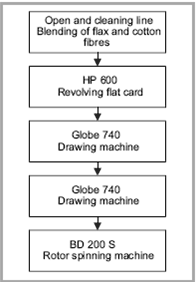Figure 1. Block-scheme of the spinning process

The following parameters were determined in order to estimate the quality parameters of yarns manufactured with the use of the BD 200S spinning machine:

v     Average linear density and its coefficient of variation,

v     Average breaking strength and its coefficient of variation,

v     Tenacity,

v     Yarn elongation at break,

v     Elasticity,

v     Average twist value and its coefficient of variation,

v     Yarn mass irregularity,

v     Number of faults per 1000 m yarn, i.e. Thin and thick places, and neps, each per 1000 m yarn, and

v     Yarn hairiness.

Only those most important parameters which have the greatest influence on yarn quality were selected for the purpose of this work and the construction of the model.

The Aim of Investigation

The main aim of the investigation was to determine the influence of the percentage content of flax in the feeding sliver, and of the linear density of the manufactured yarn on the yarn quality parameters, as well as to design an appropriate model of the spinning process.

Partial models of the spinning process were developed for selected essential yarn quality parameters, such as tenacity, yarn hairiness, and yarn faults per 1000 m. These models should permit the above-mentioned parameters in dependence of the flax percentage content in the sliver, and the linear density of the yarn manufactured to be determined. Feed-forward artificial neural networks were used for modeling. Contrary to the above formulated analysis, the publication  presents the modeling of the influence of flax content (in percentage) on the slivers mass irregularity and the sliver cohesion, as well as on the yarn mass irregularity.

Other parameters, such as average linear density and its coefficient of variation, average breaking strength and its coefficient of variation, yarn elongation at break, elasticity, average twist value and its coefficient of variation, were only measured to check the spinning process parameters, and were not used for modeling.

Selection of the Optimum Network Structure

The appropriate selection of the learning data and of the network structure is of the utmost importance in the process of modeling functional dependencies with the use of artificial neural networks. In the analysis under consideration, the measurements on whose basis the learning data vectors for the network have been constructed were designed in such a way that the particular measuring points were regularly sited throughout the measurement space. As measuring all the described yarn parameters is very laborious, their number was limited to those values which are indispensable for designing a model of the dependencies considered, and which are characterized by appropriate accuracy. A similar approach as in the previous reports [1,2] was adopted, and the complex problem of modeling the selected yarn properties was decomposed into the simpler problems of modeling each of the parameters separately. Such a method of solution is appropriate, as the dependencies of output parameters are analyzed as the functions of the same input parameters; this means dependencies on the flax percentage content (U) and the yarn linear density (Tty), and not the dependencies between the output parameters themselves. Therefore, when preparing a solution to this problem, the construction of one great neural network is not necessary, and some small networks can be designed, each of which is used to model one yarn parameter. The number of measurements which must be carried out for preparing the learning data matrix for some small networks is significantly smaller than those for one great network. However, when designing the experiment, it is necessary to remember that the number of measurements must be significantly greater than the number of weights in the network.

Feed-forward two-layer networks with a linear and a sigmoidal activation function in the hidden layer were used for the modeling of the selected yarn properties. According to literature, the directional networks are called Adaline networks, whereas networks with a sigmoidal activation function are called perceptron networks or multi-layer perceptrons (MLP) . The number of network inputs k in the network structure (k-l-m) is determined by the number of input parameters, and the number of outputs m is determined by the number of output parameters, whereas the number of neurons in the hidden layer is selected in such a way that the network would correctly model the dependencies under consideration. No exact rules or equations exist which would allow the neuron number in the hidden layer to be calculated. In general, the choice depends on the degree of the problems complexity, and on the experience of the person designing the neural network. The following criteria can be applied :

v    The neuron number in the hidden layer should be relatively great in order to obtain the desired approximation accuracy of the functional dependencies modelled,

v    The neuron number in the hidden layer should not be too great, so that the network does not lose its generalizing abilities as well as to avoid the effect of modeling the measurement uncertainty of the quantities recorded.

In our case, the MLP networks with (2-l-1) structure were used to model the selected yarn parameters. The selection of the optimal neuron number l in the hidden layer was verified experimentally for each of the tested yarn parameters on the basis of computer simulations carried out. For each of the analyzed cases, the (2-3-1) structure was selected as the best.

The mean square errors cost function and the Levenberg-Marquardt optimisation algorithm were used for the networks training . Model verification was performed by the use of the leave-one-away method .

Modelling of Yarn Tenacity

The measurement results of yarn tenacity Ww for different percentages of flax content U, and yarn linear density Tt, for both variants W1 and W2, are presented in Figure 2. At the first stage of modelling, the dependence of yarn tenacity on the yarn linear density was approximated by the following linear functions:

Ww = a1 Tt + a0 (1)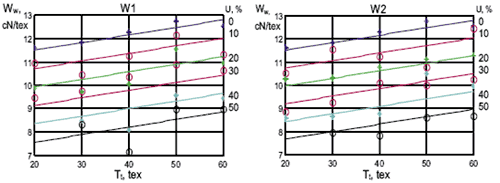Figure 2. Approximation of the dependence of yarn tenacity Ww on the flax percentage content U and yarn linear density Tt for the W1 and W2 variants by means of the Adaline networks.

For different values of the parameter U. The values of the coefficients a1 and a0 of function (1) were determined experimentally by means of the least-squares method for the variants W1 and W2, and for each of the values of the parameter U tested. The mean square errors of approximation by means of the straight lines family equalled S1=0.078 cN/tex for the W1 variant and S2=0.079 cN/tex for the W2 variant.

The straight-line families determined for the variants W1 and W2 approximate the dependency of yarn tenacity on its linear density for different percentages of flax content, which is present as a parameter in the model. A disadvantage of these models is the fact that the models allow calculation of the yarn tenacity only for those values of the parameter U tested for which the coefficients of function (1) were determined. This disadvantage can be eliminated by substituting the above-described models with artificial neural networks (ANN).

An approximation of the dependencies discussed by means of an Adaline-type network is shown in Figure 2. The networks used are feed-forward networks, with a linear activation function in every layer. Two-layer networks with a (2-3-1) structure were used for modelling. This means that the network has two inputs, three neurons in the hidden layer, and one neuron in the output layer. The mean square errors of approximation for this case equals S1=0.091 cN/tex for the W1 variant and S2=0.088 cN/tex for the E2 variant. They are only insignificantly higher than for the approximation by the straight lines family.

An approximation of the dependence of yarn tenacity Ww on the flax percentage content U and the yarn linear density Tt by means of a multi-layer perceptron type network of a (2-3-1) structure is presented in Figure 3. The network has two inputs, three neurons with sigmoidal activation function in the hidden layer and one linear neuron in the output layer. The mean square errors of approximation for this case equals S1=0.059 cN/tex for the W1 variant and S2=0.074 cN/tex for the W2 variant.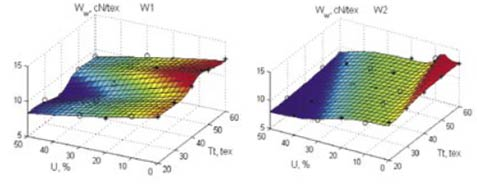Figure 3. Approximation of the dependence of yarn tenacity Ww on flax percentage content U and yarn linear density Tt by means of a multi-layer perceptron type network for the W1 and W2 variants (3D presentation).

Similar results were obtained for both variants W1 and W2 with the use of feed-forward two-layer networks with a (2-3-1) structure, when neurons with a hyperbolic tangent function were applied in the hidden layer instead of the sigmoidal activation function.

Approximation by the use of the MLP networks ensured higher approximation accuracy, but the models obtained by the use of the Adaline network have better generalisation properties, and the values of approximation errors are still satisfactory.

Modelling Yarn Hairiness

The yarn hairiness H measurement results for different values of flax percentage content U and yarn linear density Tt for the W1 and W2 variants are presented in Figure 4. The yarn hairiness was measured by an Uster Tester 3 apparatus. The mean square errors of approximation by means of the MLP networks with a (2-3-1) structure equals S1=0.01 cN/tex for the W1 variant and S2=0.013 cN/tex for the W2 variant.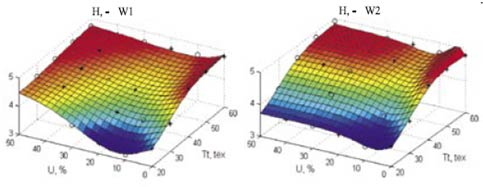Figure 4. Dependence of the yarn hairiness H on the flax percentage content U and yarn linear density Tt for the W1 and W2 variants, together with its approximation by MLP networks with a (2-3-1) structure; hairiness measured by an Uster Tester 3 apparatus.

The yarn hairiness H measurement results of yarns with different values of flax percentage content U and yarn linear density Tt for the W1 and W2 variants, measured by a Shirley apparatus at the distance of 3 mm, are presented in Figure 5. The mean square errors of approximation by means of the MLP networks with (2-3-1) structure equals S1=0.07 cN/tex for the W1 variant and S2=0.06 cN/tex for the W2 variant.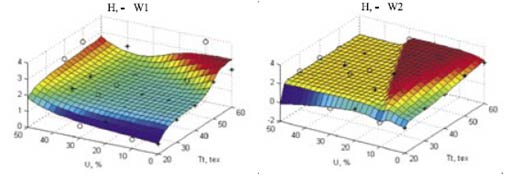Figure 5. Dependence of the yarn hairiness H on the flax percentage content U and yarn linear density Tt for the W1 and W2 variants, together with its approximation by MLP networks with a (2-3-1) structure; hairiness measured by a Shirley apparatus.

Modelling the Number of Yarn Faults

1.       Number of thin places

The number of yarn thin places p measured for different values of flax percentage content U and yarn linear density Tt for the W1 and W2 variants are presented in Figure 6. The mean square errors of approximation by means of the MLP networks with a (2-3-1) structure equals S1=2.75 per 1000 m for the W1 variant and S2=1.7 per 1000 m for the W2 variant.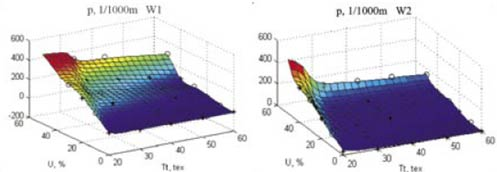Figure 6. Dependence of the number of yarn thin places p on the flax percentage content U and yarn linear density Tt for the W1 and W2 variants, together with its approximation by MLP networks with a (2-3-1) structure.

1.       Number of thick places

The number of yarn thick places z measured for different values of flax percentage content U and yarn linear density Tt for the W1 and W2 variants are presented in Figure 7. The mean square errors of approximation by means of the MLP networks with a (2-3-1) structure equals S1=7.6 per 1000 m for the W1 variant and S2=5.2 per 1000 m for the W2 variant.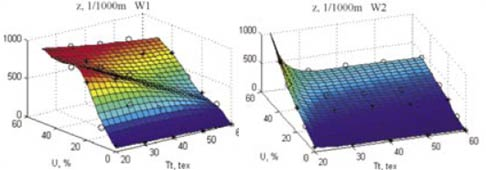Figure 7. Dependence of the number of yarn thick places z on the flax percentage content U and yarn linear density Tt for the W1 and W2 variants, together with its approximation by MLP networks with a (2-3-1) structure.

2.       Number of neps

The number of neps n measured for different values of flax percentage content U and yarn linear density Tt for the W1 and W2 variants are presented in Figure 8. The mean square errors of approximation by means of the MLP networks with a (2-3-1) structure equals S1=7.5 per 1000 m for the W1 variant and S2=2.0 per 1000 m for the W2 variant.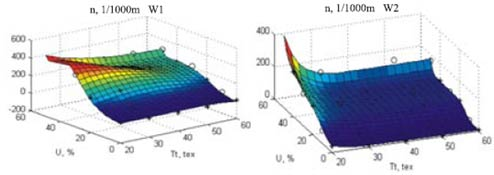Figure 8. Dependence of the number of neps n on the flax percentage content U and yarn linear density Tt for the W1 and W2 variants, together with its approximation by MLP networks with a (2-3-1) structure.

Conclusions

v     Blended flax/cotton yarns in which carded flax was used (variant W2) show better quality parameters as yarns of the variant W1.

v     Blended yarns have lower tenacity when compared with 100% cotton yarn. The tenacity of blended yarns decreases with the increase in the blends flax content. The partial models described in the paper allow the prediction of yarn tenacity for different yarn linear densities and flax percentage contents.

v     The number of faults, i.e. of thin & thick places and of neps, is the lowest for pure cotton yarn. The number of faults increases non-linearly with the increase in flax content in the blend. Quality and quantity analyses of yarn faults were presented in the paper.

v    The hairiness increases with the increase in the yarns linear density. We could not unambiguously define the influence of the fibre composition in the blended yarns on yarn hairiness.

v     The research results concerning the selected yarn properties served for the development of a model of the spinning process designed by means of artificial neural networks (ANN). This model allows the determination of selected yarn parameters on the basis of yarn linear densities, flax percentage content and the method of blended sliver preparation (variant W1 or W2) for the yarns produced on the BD 200S rotor spinning machines.

References

1. Jackowska-Strumiłło L., Jackowski T., Chylewska B., Cyniak D.: Application of Hybrid Neural Model to Determination of Selected Yarn Parameters, Fibres and Textiles in Eastern Europe, 1998, No. 4, p. 27-32.
2. Jackowski T., Chylewska B., Cyniak D., Czekalski J., Jackowska-Strumiłło L.: Modelling of the relationship between feeding sliver structures and parameters of cotton/linen blended yarns, Fibres & Textiles in Eastern Europe, April/June 2003,Vol. 11, No. 2 (41), p. 12-17.
3. Jackowski T., Czekalski J., Chylewska B., Cyniak D.: Blended rotor yarns with cottonised hemp content (in Polish), Przegląd Włkienniczy, 7/2001.
4. Tadeusiewicz R.: Sieci Neuronowe. PWN, Warszawa 1991.
5. Haykin S.: Neural Networks: a comprehensive foundation - 2nd ed. Prentice Hall, 1999.
6. Cierpucha W., Kozłowski R., Mańkowski J., Waśko J., Mańkowski T.: Applicability of Flax and Hemp as Raw Materials for Production of Cotton-like Fibres and Blended Yarns in Poland, Fibres & Textiles in Eastern Europe, July/October 2004, Vol. 12, No. 3 (47), p. 13-18.
7. Hecht-Nielsen R., Neurocomputing. Addison-Wesley Publishing Company, 1990